Question

The face value of a simple discount note is \$4,000. The bank discount is calculated at...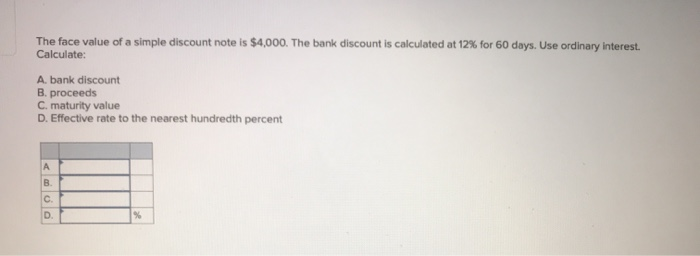The face value of a simple discount note is \$4,000. The bank discount is calculated at 12% for 60 days. Use ordinary interest. Calculate: A bank discount B. proceeds C. maturity value D. Effective rate to the nearest hundredth percent

Ordinary Interest = I. = Pr (D/360); I. = 4000 x 60/360 x 12/100; I. = \$80

A, Bank discount = (face value)(discount rate)(time) = (4,000)(0.12)(60/360) = 28800/360 = \$ 80.00

B, Proceeds = face value-bank discount = 4,000-80 = \$ 3,920

C, MV = P * ( 1 + r )n

MV = 4000 * (1+12%)0.164384

MV = 4000 * 1.524454491 = \$ 6097.817964

D, it = (1 + i)t - 1

it = (1 + 0.0722901)5 - 1 = 0.417625 = 41.76%

And we would also get it = ( 1 + ( r / m ) )mt - 1 = 41.76%

Earn Coins

Coins can be redeemed for fabulous gifts.

Similar Homework Help Questions
• Bill Blank signed an \$7,540 note at Citizen's Bank. Citizen's charges a 8.2% discount rate. Assume...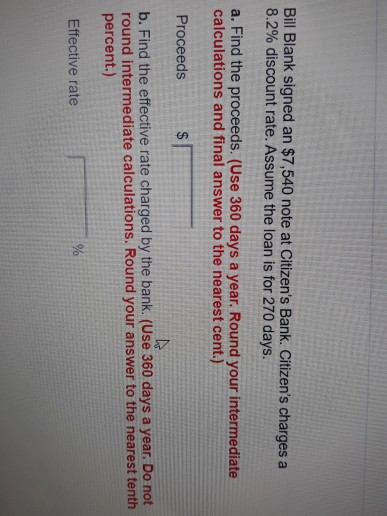Bill Blank signed an \$7,540 note at Citizen's Bank. Citizen's charges a 8.2% discount rate. Assume the loan is for 270 days. a. Find the proceeds. (Use 360 days a year. Round your intermediate calculations and final answer to the nearest cent.) Proceeds b. Find the effective rate charged by the bank. (Use 360 days a year. Do not round intermediate calculations. Round your answer to the nearest tenth percent.) Effective rate You were offered the opportunity to purchase either...

• Sam Peters needs to calculate the effective interest rate of a simple discount note for \$3,600,...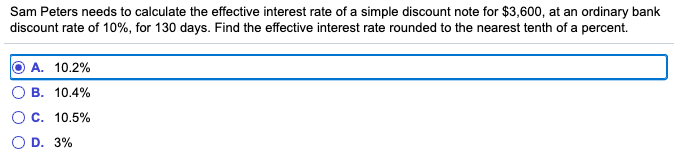Sam Peters needs to calculate the effective interest rate of a simple discount note for \$3,600, at an ordinary bank discount rate of 10%, for 130 days. Find the effective interest rate rounded to the nearest tenth of a percent. O A. 10.2% OB. 10.4% O C. 10.5% OD. 3%

• The face value of both a simple interest and a simple discount note are \$6,000. If...

The face value of both a simple interest and a simple discount note are \$6,000. If both notes have interest rates of 8% for 90 days, calculate: a. Amount of interest charged for each type of note. b. Amount each borrower would receive. c. Amount payee would receive at maturity.

• A man has a simple discount note for ​\$6,200​, at an ordinary bank discount rate of...

A man has a simple discount note for ​\$6,200​, at an ordinary bank discount rate of 8.53%, for 50 days. What is the effective interest​ rate using the banker's rule?

• Solve for maturity value, discount period, bank discount, and proceeds.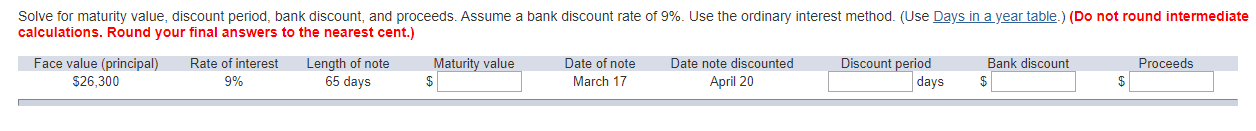Solve for maturity value, discount period, bank discount, and proceeds. Assume a bank discount rate of 9%. Use the ordinary interest method. (Use Days in a year table.) (Do not round intermediate calculations. Round your final answers to the nearest cent.)

• Complete the following table for the simple discount notes. Use the ordinary interest method. (Use 360...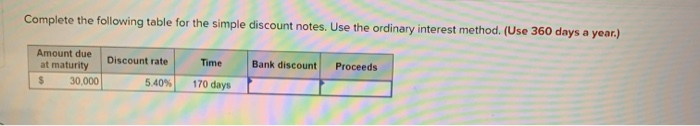Complete the following table for the simple discount notes. Use the ordinary interest method. (Use 360 days a year.) Bank discount Proceeds Amount due at maturity 30.000 Discount rate 5.40% Time 170 days

• 2) Marcia Rodger borrowed \$3,500 from Valley Bank at a rate of 9 %. The date of the loan was October 10. Marcia hop...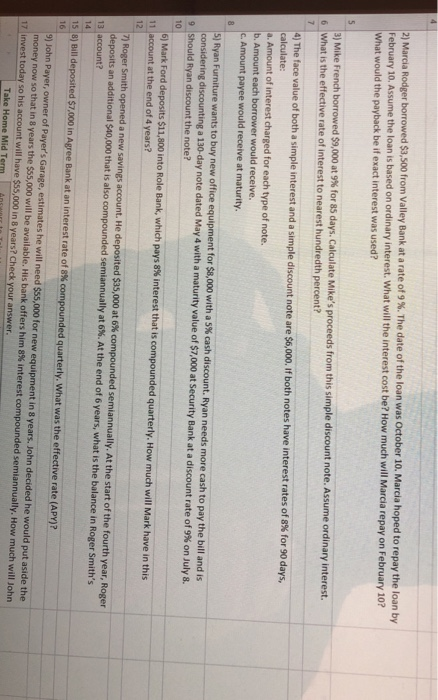2) Marcia Rodger borrowed \$3,500 from Valley Bank at a rate of 9 %. The date of the loan was October 10. Marcia hoped to repay the loan by February 10. Assume the loan is based on ordinary interest. What will the interest cost be? How much will Marcia repay on February 10? What would the payback be if exact interest was used? 3) Mike French borrowed \$9,000 at 9% for 85 days. Calculate Mike's proceeds from this simple discount...

• Use ordinary interest: Principal \$70,000 Rate of Interest: 11% Time: 90 days Maturity Value: A Date note made Mar 10 Date note discounted: April 15 discount period: B proceeds: C note to be discounted at 10%

Use ordinary interest: Principal \$70,000 Rate of Interest: 11% Time: 90 days Maturity Value: A Date note made Mar 10 Date note discounted: April 15 discount period: B proceeds: C note to be discounted at 10%

• On May 7, Ralph Blue accepted a \$5,000 note from Dick Shea. Terms of the note...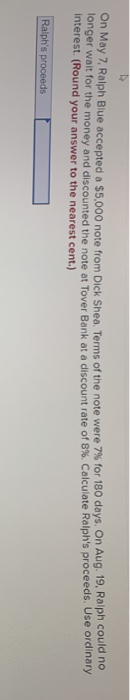On May 7, Ralph Blue accepted a \$5,000 note from Dick Shea. Terms of the note were 7% for 180 days. On Aug. 19, Ralph could no onger walt for the money and discounted the note at Tover Bank at a discount rate of 8 %. Calculate Ralph's proceeds. Use ordinary interest. (Round your answer to the nearest cent.) Ralph's proceeds

• Accounting or Bonds Sold at a Discount The Biltmore National Bank raised capital through the sale...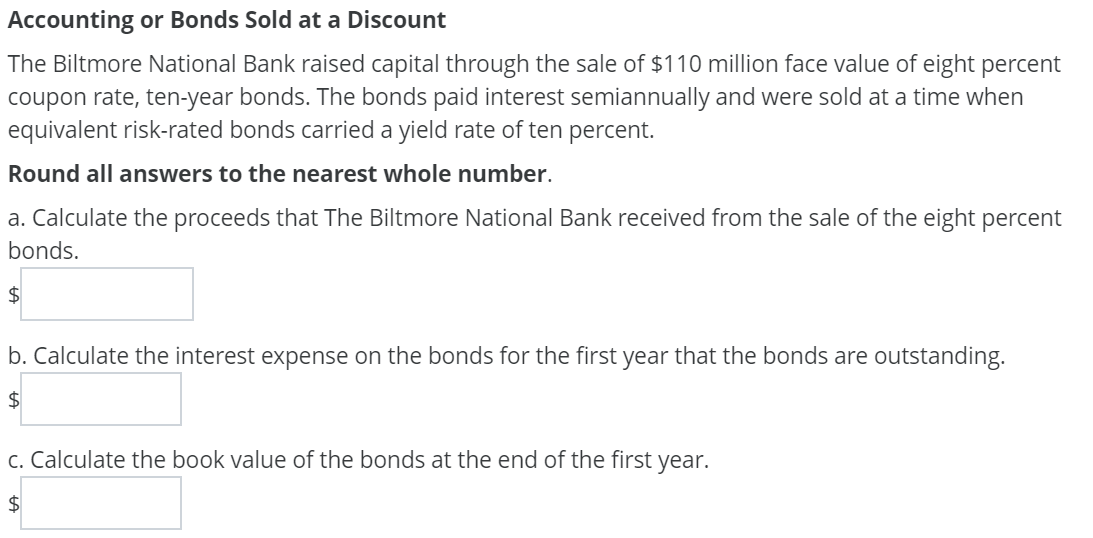Accounting or Bonds Sold at a Discount The Biltmore National Bank raised capital through the sale of \$110 million face value of eight percent coupon rate, ten-year bonds. The bonds paid interest semiannually and were sold at a time when equivalent risk-rated bonds carried a yield rate of ten percent. Round all answers to the nearest whole number. a. Calculate the proceeds that The Biltmore National Bank received from the sale of the eight percent bonds. \$ b. Calculate the...